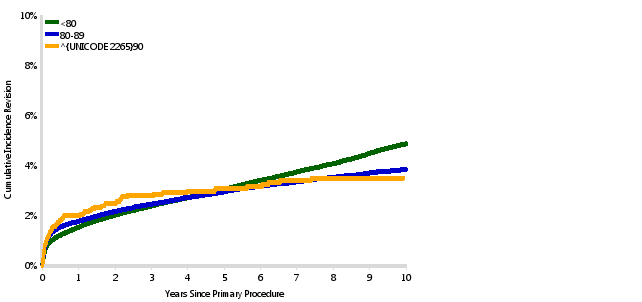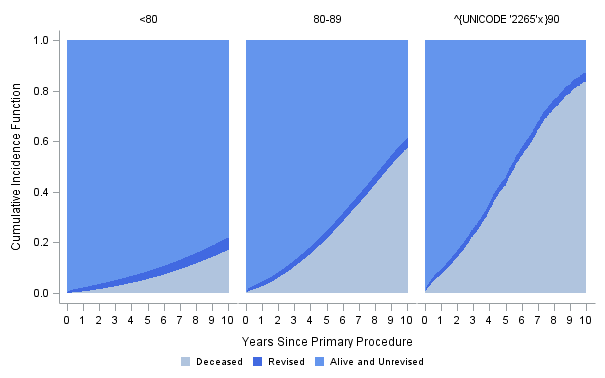## Unicode issues in the graph

I did asked about the unicode issue in proc gplot and proc sgplot. However, both just temporarily solved. I know annotation can fix the issue but not familiar with it. Anyone can help me about this? Further, anyone can give me some information about how to set up annotation data?

Two graphs.

First use proc gplot:

``````proc format;
value ageold		1="<80"
2="80-89"
3="^{UNICODE 2265}90"
999999="TOTAL"
;
run;

proc gplot data=summary3 gout=hazgraph;

format ageold ageold.;

plot y*time = ageold / legend=legend1 haxis=axis1 vaxis=axis2 noframe;

run;

quit;``````The second use sgpannel. Still not working

``````ods escapechar="^";
ods graphics on / noborder width=16cm height=10cm outputfmt=emf nosubpixel;
proc sgpanel data=summary3 nosubpixel;
format ageold ageold.;
where years<=10;
band x=years lower=0 upper=deceased / legendlabel="Deceased" ;
band x=years lower=deceased upper=revised / legendlabel="Revised";
band x=years lower=revised upper=alive / legendlabel="Alive and Unrevised";
rowaxis label="Cumulative Incidence Function"; colaxis label="Years Since Primary Procedure" values=(0 to 10 by 1);
keylegend / noborder autooutline;
run;
ods graphics off;``````

The graph is3 REPLIES 3Jay54
Meteorite | Level 14

## Re: Unicode issues in the graph

When using Unicode in the Format, you need to use the default ODS escape character string (*ESC*).  The value should be:

3 = "(*ESC*){unicode '2265'x} 90"

See example:

https://blogs.sas.com/content/graphicallyspeaking/2015/07/29/unicode-in-formatted-data-sas-9-40m3/

## Re: Unicode issues in the graph

Thanks! sgpannel works well but gplot is still not working. I suppose annotation is needed for proc gplot.

## Re: Unicode issues in the graph

You might try Dr. Robert Allison's solution in this post:

How to use unicode >= Symbol in SAS/Graph Legend

Using that approach, I think your format would be:

``````proc format;
value ageold 1='003C00380030'x
2='00380030002D00380039'x
3='226400390030'x
999999='0054004F00540041004C'x
;
run;``````

In your LEGEND statement, be sure to specify a Unicode font as shown in this example:

``legend1 label=('Age: ') value=(justify=left font='albany amt/unicode');``
Discussion stats
• 3 replies
• 1390 views
• 1 like
• 3 in conversation